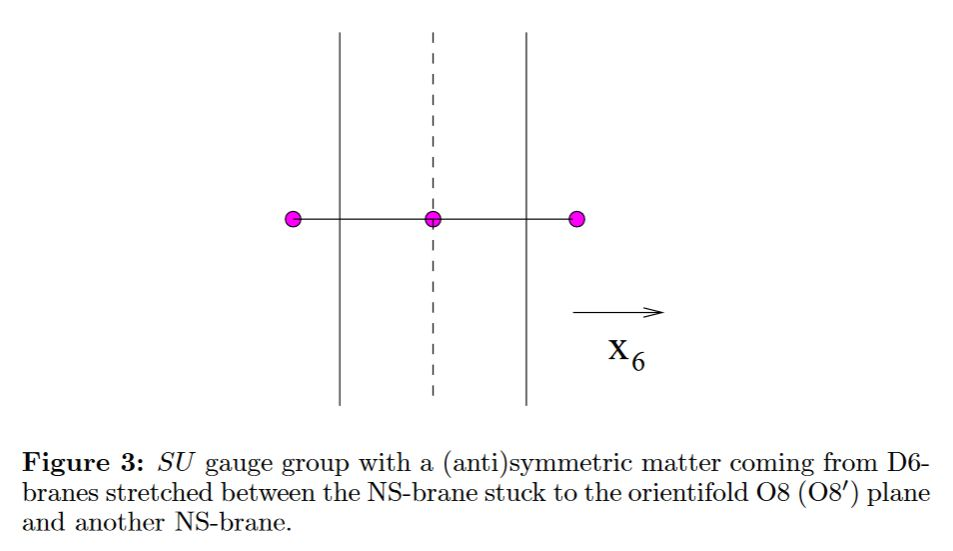Contents

# Contents

## Idea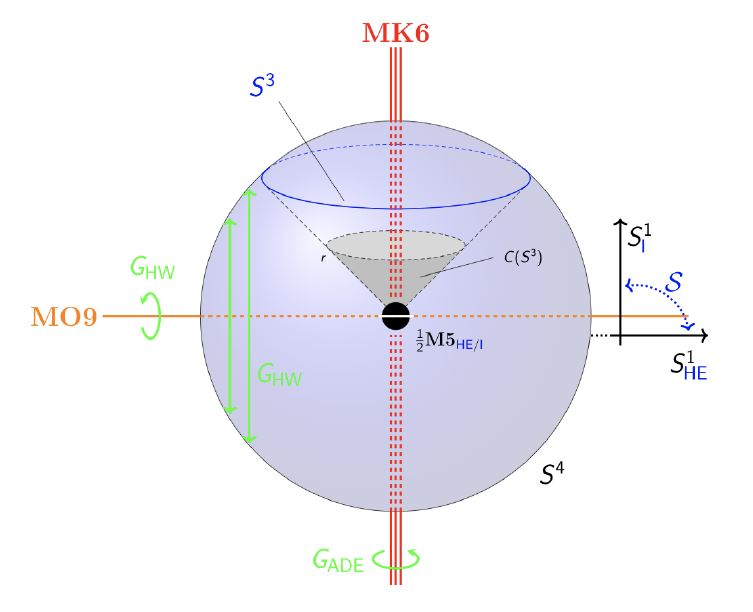$S^1 \!\sslash\! G_{HW} \times \mathbb{H}\!\sslash\! G_{ADE}$

which are, locally, the Cartesian product of

1. the circle orientifolded by $G_{HW} \simeq \mathbb{Z}_2$ as in Horava-Witten theory;

2. the quaternions orbifolded by a finite subgroup of SU(2) $G_{ADE}$.

graphics grabbed from HSS18, Example 2.2.7

Depending on whether the circle fiber for duality between M-theory and type IIA string theory is taken to be along the orbit of $G_{HW}$ or of $\mathbb{Z}_{n} \subset G_{ADE}$ this is

For $G_{ADE} = \mathbb{Z}_2$ this subsumes M-theory on K3 times $S^1 \sslash G_{HW}$ (Seiberg-Witten 96)

## Relation to $D = 6$$N=(1,0)$ SCFT

Gives rise to D=6 N=(1,0) SCFT ( DHTV 14, Section 6, Gaiotto-Tomasiello 14, HKLY 15)

## Dual perspectives

(…)

### Type I’ with D6s ending on $\tfrac{1}{2}$ NS5-branes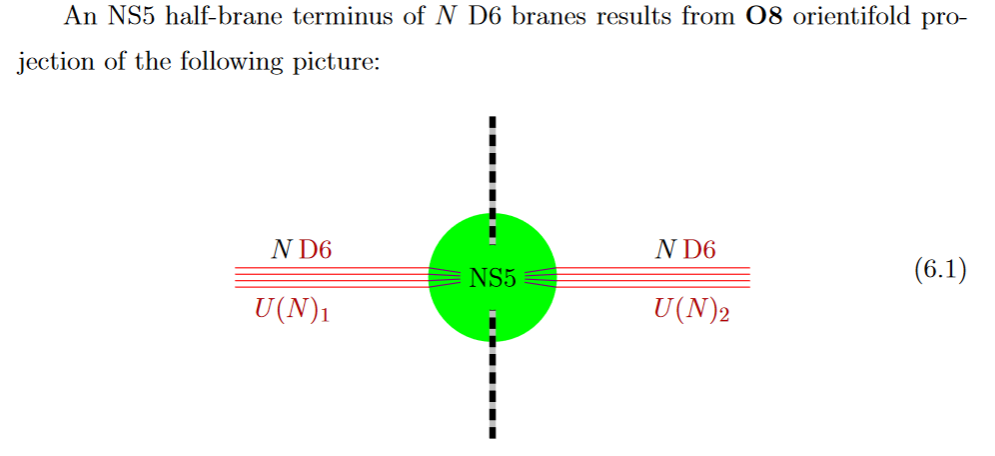from GKSTY 02

If in addition the black NS5-brane sits at an O8-plane, hence at the orientifold fixed point-locus, then in the ordinary $\mathbb{Z}/2$-quotient it appears as a “half-brane” with only one copy of D6-branes ending on it: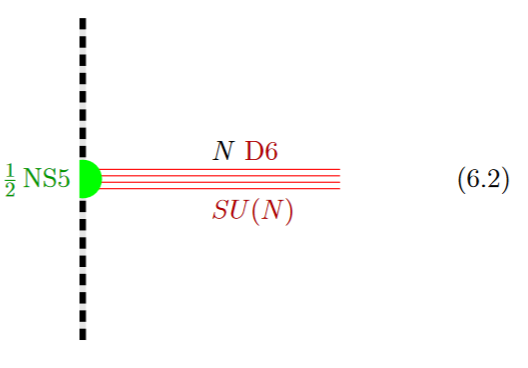from GKSTY 02

(In Hanany-Zaffaroni 99 this is interpreted in terms of the 't Hooft-Polyakov monopole.)

The lift to M-theory of this situation is an M5-brane intersecting an M9-brane (see at M-theory on S1/G_HW times H/G_ADE):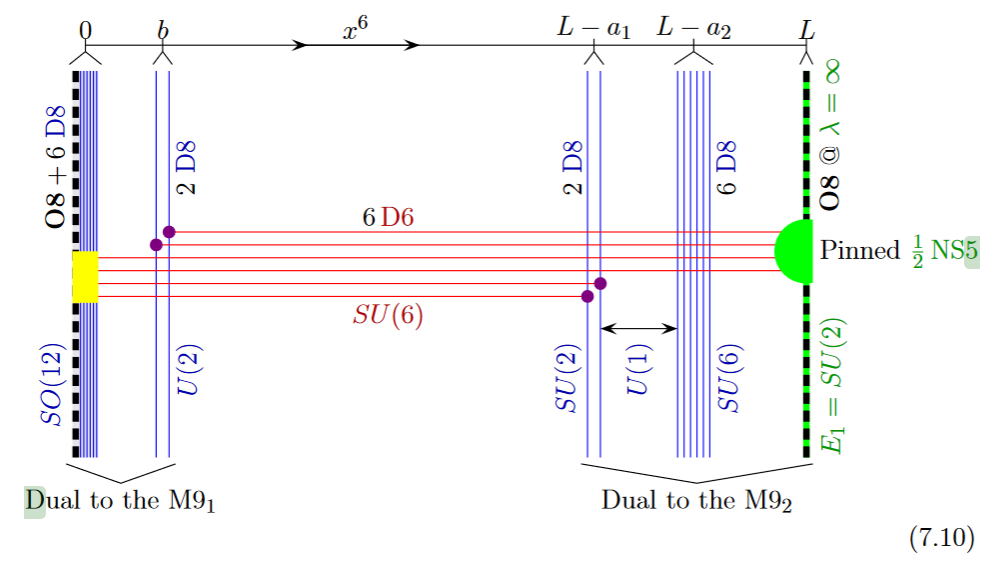from GKSTY 02

Alternatively the O8-plane may intersect the black D6-branes away from the black NS5-brane: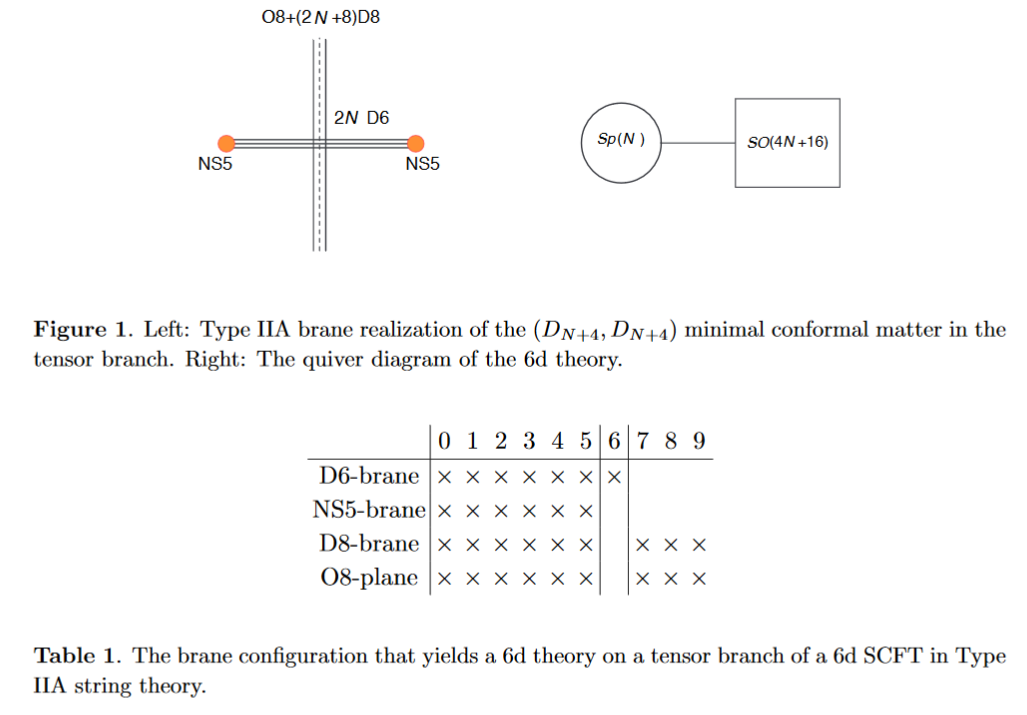from HKLY 15

In general, some of the NS5 sit away from the O8-plane, while some sit on top of it: